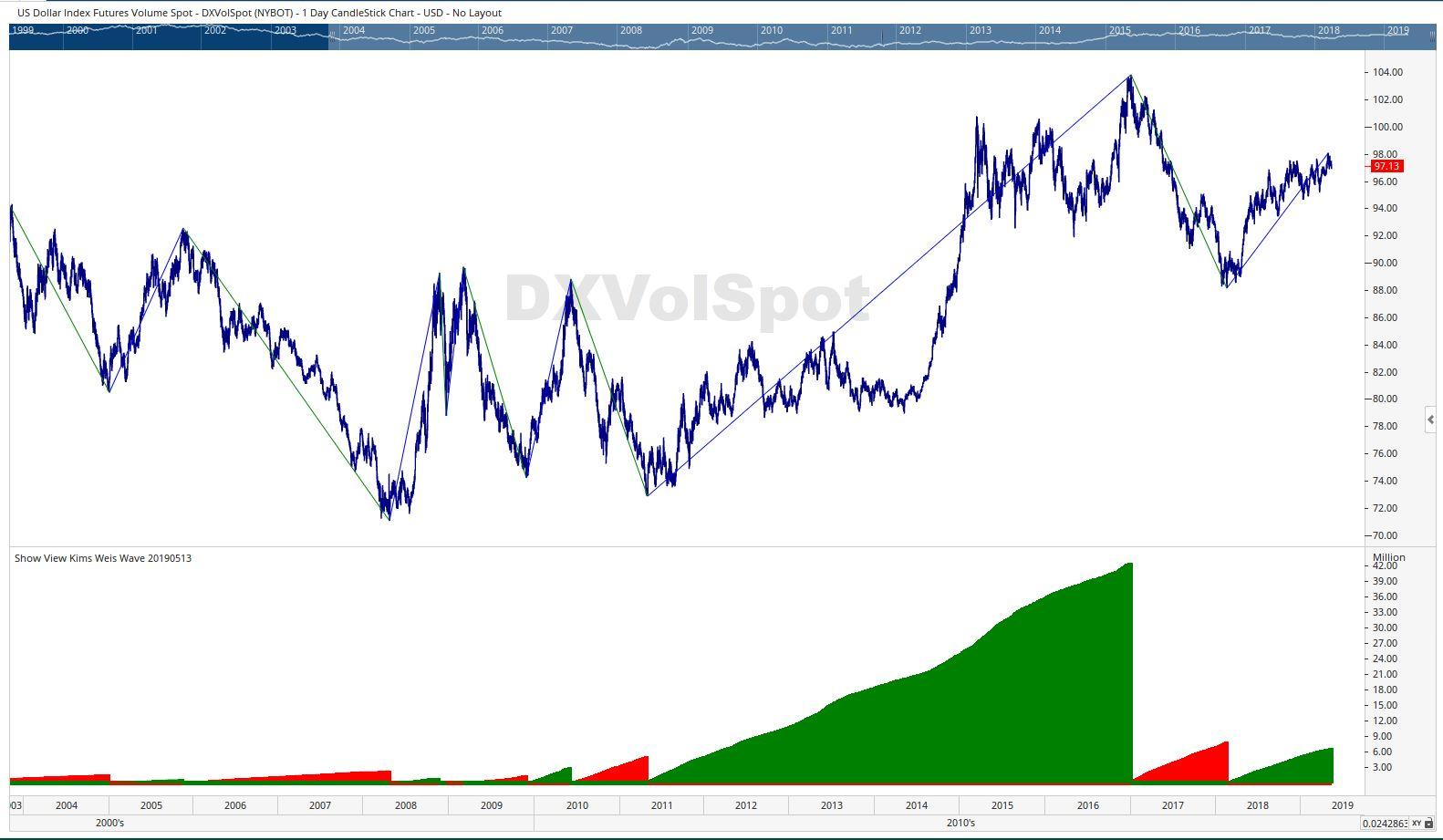Select Page

# Reply To: Variant Of Weis Wave

Optuma Forums Optuma Scripting Variant Of Weis Wave Reply To: Variant Of Weis Wave

#53035

Hi Kim & Shannon,

Kim, your Percent Plot on the chart is 5% and your script 10%. Always make sure they are the same

Here is a minimalist listing of the script, use Notepad to copy/paste and replace the “em dash” with a hyphen “-” (as suggested by Matthew) when using this script:

// Kim’s Weis Wave 20190513

PSW = PERCENTSWING(PERCENT=10, DEFAULT=SwingList, COLOURSTYLE=Up/Down);

PSWStart= SWINGSTART(PSW);

PSWEnd = SWINGEND(PSW);

Rng = PSWStart – PSWEnd;

Sig1 = if((TIMESINCESIGNAL(PSWStart) > 0 and Rng > 0) , 1, 0);

Sig2 = IF(TIMESINCESIGNAL(PSWStart) > 0 and Rng < 0, -1, 0) ;

// Plot the Down trend Volume

Plot1 = If(Sig1, ACCSINCESIGNAL(Vol(), Sig1), 0) ;

Plot1.Colour = Red;

Plot1.Plotstyle = Histogram;

Plot1.LineWidth = 5;

Plot1.LineStyle = Solid;

// Plot the Up trend Volume

Plot2 = If(Sig2, ACCSINCESIGNAL(Vol(), Sig2), 0) ;

Plot2.Colour = Green;

Plot2.Plotstyle = Histogram;

Plot2.LineWidth = 5;

Plot2.LineStyle = Solid;

Here a screenshot with both the script and Percent Swing Overlay Plot set for 10%:Cheers

Trevor

The Auld Tyma from